## Example Questions

### Example Question #1 : How To Find An Angle In A Quadrilateral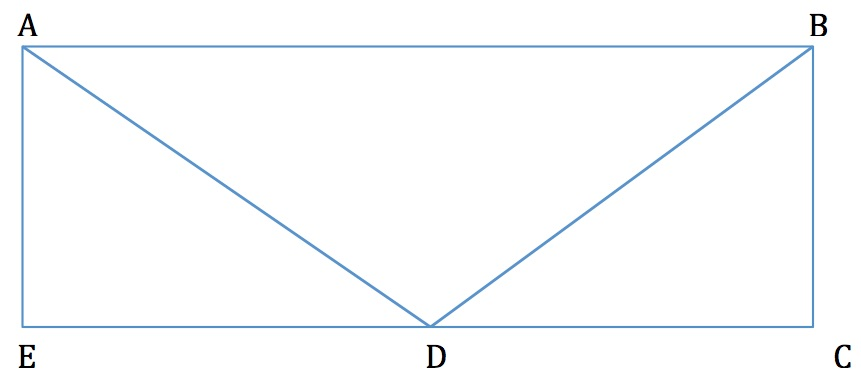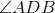bisects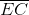. If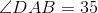then, in degrees, what is the value of?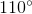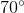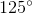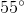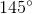Explanation:

A rectangle has two sets of parallel sides with all angles equaling 90 degrees.

Sincebisectsinto two equal parts, this creates an isosceles triangle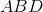.

Therefore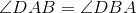. The sum of the angles in a triangle is 180 degrees.

Therefore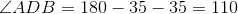### Example Question #2 : How To Find An Angle In A Quadrilateral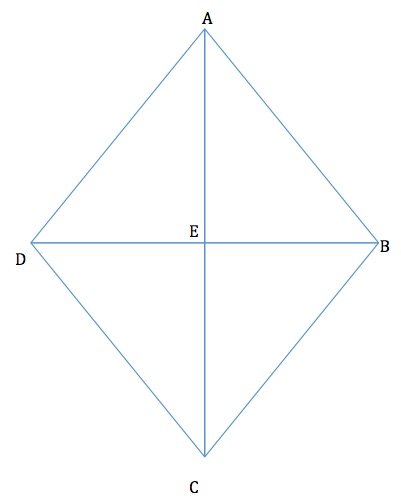The rhombus above is bisected by two diagonals.

If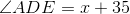and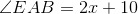then, in degrees, what is the value of the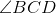?

Note: The shape above may not be drawn to scale.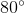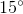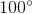Explanation:

A rhombus is a quadrilateral with two sets of parallel sides as well as equal opposite angles. Since the lines drawn inside the rhombus are diagonals,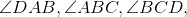and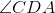are each bisected into two equal angles.

Therefore,, which creates a triangle in the upper right quadrant of the kite. The sum of angles in a triangle is 180 degreees.

Thus,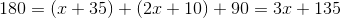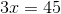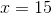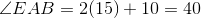Since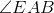is only half of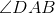,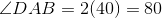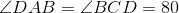### Example Question #3 : How To Find An Angle In A Quadrilateral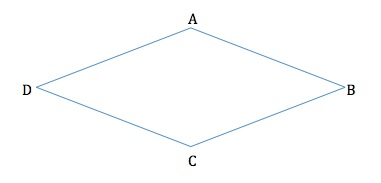If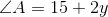and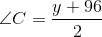, then, in degrees, what is the value of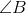?

Note: The figure may not be drawn to scale.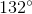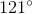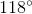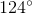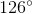Explanation:

In a rhombus, opposite angles are equal to each other. Therefore we can set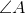and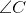equal to one another and solve for: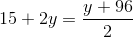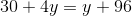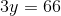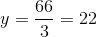Therefore,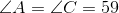A rhombus, like any other quadrilateral, has a sum of angles of 360 degrees.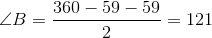### Example Question #4 : How To Find An Angle In A Quadrilateral

The interior angles of a quadrilateral are, and. What is the measure of the smallest angle of the quadrilateral?Explanation:

In order to solve this problem we need the following key piece of knowledge: the interior angles of a quadrilateral add up to 360 degrees. Now, we can write the following equation:When we combine like terms, we get the following:We will need to subtract 71 from both sides of the equation:Now, we will divide both sides of the equation by 17.We now have a value for the x-variable; however, the problem is not finished. The question asks for the measure of the smallest angle. We know that the smallest angle will be one of the following:orIn order to find out, we will substitute 17 degrees for the x-variable.Because 51 degrees is less than 71 degrees, the measure of the smallest angle is the following: